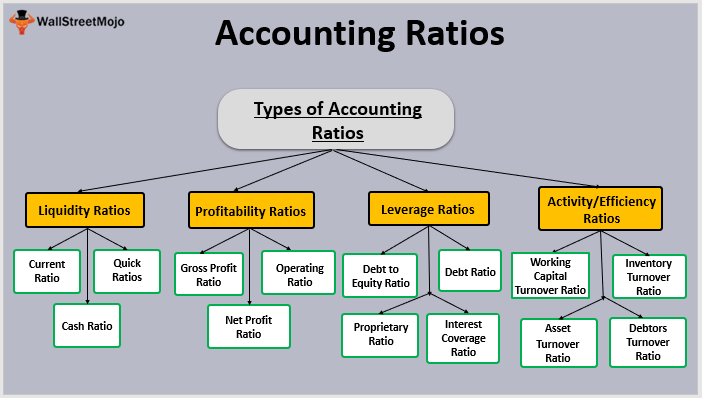# Introduction to Accounting Ratios

Welcome to class!

In today’s class, we will be talking about the introduction to accounting ratios. Enjoy the class!

### Introduction to Accounting Ratios#### Margin and Mark Up

The ability to calculate margin and mark up may be necessary to solve some incomplete record problems.

##### MARGIN

When gross profit is expressed as a fraction or percentages of selling price it is known as margin.

Example:                                                                              N

The cost price of goods                                                          100

Profit                                                                                        25

Selling price                                                             125

Then the margin    = profit   x    100 = 25 x 100 = 20% 0r 1/5

Selling price     1      125    1

##### MARK-UP

When gross profit is expressed as a percentage or fraction of the cost of sales is known as mark – up.

In the above example, mark – up is           profit                                 x 100   =  25% or ¼

Cost of goods sold                 1

##### Relationship between margin and mark – up

Examples:

If mark – up                                                                                                    Margin

Is (i) 2                                                    then                                       2         = 2

5                                                                                                     5 +  2     7

(ii)          1                                              then                                       1           = 1

4                                                                                              4+ 1        5

If margin                                                                                 mark -up

Is            (i)  2                                        then                              2          = 2

5                                                                             5  – 2          3

(ii) 1                                        then                           1         = 1

3                                                                             3– 1        2

(iii) 1                                        then                           1         = 1

7                                                                            7 – 1       6

NB:- making-up is always greater than the margin

FURTHER EXAMPLES

1. Cost of sales: N3000. Margin 25%. Calculate sales revenue.

Solution: margin is 1 therefore Mark-up = 1      = 1

4                                      4 -1       3

N 3000 X 1 = PROFIT = N1000

3

Therefore Sales revenue = cost + profit = N (3000 + 1000)

= N 4000

NB: it is mark-up that is a percentage of cost hence the margin given in the question must be converted to mark-up to solve the above problem.

1. Sales revenue: N7000. Make-up is 40%. Calculate the gross profit.

Solution: mark – up; is 2, therefore, Margin is  2      =  2

5                                     5 + 2    7

Therefore Gross profit = 2 x N7000 = N2000

7

1. Maheen provides the following information for the year ended 31st 2003.

N

Stock at 1st Jan. 2003                                                          9000

Stock at 31st Dec. 2003                                                       11,000

Sales in the year ended 31st                                              84,000

Maheen sells her goods at a make – up of 331/3. Prepare Maheen’s trading account for the year ended 31st Dec. 2003 in as many details as possible.

Solution: This is a good example of a problem that is solved by working backwards

MAHEEN

Trading account for the year ended 31 Dec. 2003

N             N

Sales (given)                                                                                                   84,000

Less cost of sales

Opening stock 1st Jan (given)                                                    9000

Step 4 purchases (balance figure 3)                                        65000

Step 3 Gods available for sales (balancing figure 2)                      74000

Less closing stock at 31 Dec. (given)                            11000

Step 2 cost of sales (balancing fig 1)                                                     63,000

Step 1 gross profile (1/4 x N84,000)                                                           21,000

iii.         STOCK LOST IN FIRE OR BY THEFT

The methods used for preparing account from incomplete records are also used to calculate the value of stock lost in a fire or by theft when detailed stock records have not been kept, or have been destroyed by fire.

You can solve this type of problem by preparing a pro forma “trading Account’ (It is described as “pro forma because it is not prepared like a normal trading account by transferring balances from ledger accounts.)

EVALUATION

1. Define the following accounting terms

(a) Mark-up   (b) Margin

1. State the relationship between mark-up and margin.

Example

Uwa’s warehouse was burgled on 10th April 2004. the thieves stole most of the stock but left goods worth N1250. Uwa supplies the following information:

Extracts from Uwa’s Balance Sheet at 31 Dec. 2003

Stock                                                                                      N 30,000

Debtors                                                                                 N 40,000

Creditors                                                                               N 20,000

Extracts from cash Book, 31 Dec. 2003 to 10 April 2004

Receipts from debtors                                                      N 176,000

Payments to suppliers                                                       N 120,000

Other information

Debtors at 10th April 2004                                                 N 24000

Creditors at 10th April, 2004                                             N 26,000

Uwa sells his goods at a mark-up of 25%

Required: calculate the cost of the stolen goods

Solution:                                            UWA

Pro forma Trading Account for the period 1st January, to 10th April 2004

N                     N

Sales (see wk 1 below)                                                                 160,000

Less cost of sales

Stock 1st Jan. 2004                                                 30,000

Purchases (see wk 2 below)                               126,000

156,000

Less closing 10/4/04

(Balancing figure)                                                  28,000

Cost of sales                                                                        128,000

Gross profit (mark-up is 25% so margin                                    32,000

Is 20%, (N160,000 x 20%

Therefore Cost of stock stolen = N(28,000 – 12500 = N26,750 i.e. closing stock – stock left after burglary.

Workings (1)                                    Debtors Control A/C

N                                                                     N

1 Jan. Balance b/f                         40,000                        10 April Cash           176,000

10 April Sales                                                                        Debtors out-

(balanced fig)                                160,000                      standing                   24,000                                                                                    200,000                                                          200,000

Workings (2)                        Creditors Control A/C

N                                                                     N

1 Jan. Bal b/f                       20,000

10 April, cash                                   120,000          10 April purchases

(balancing fig.)                  126,000

146,000                                                          146,000

###### GENERAL EVALUATION
1. State five features of capital expenditure
2. State five characteristics of a depreciable asset
3. List five advantages and three disadvantages of the straight line method of calculating depreciation
4. List ten users of financial accounting information
5. List six causes of depreciation of fixed assets

In our next class, we will be talking about Control Account.  We hope you enjoyed the class.

Should you have any further question, feel free to ask in the comment section below and trust us to respond as soon as possible.

Pass WAEC, JAMB, NECO, BECE In One Sitting HERE!